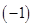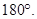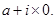# NCERT Solutions class-11 Maths Exercise 14.1## myCBSEguide App

CBSE, NCERT, JEE Main, NEET-UG, NDA, Exam Papers, Question Bank, NCERT Solutions, Exemplars, Revision Notes, Free Videos, MCQ Tests & more.

Exercise 14.1

1. Which of the following sentences are statements? Give reason for your answer.

(i) There are 35 days in a month.

(ii) Mathematics is difficult.

(iii) The sum of 5 and 7 is greater than 10.

(iv) The square of a number is an even number.

(v) The sides of a quadrilateral have equal length.

(vii) The product ofand 8 is 8.

(viii) The sum of all interior angles of a triangle is(ix) Today is a windy day.

(x) All real numbers are complex numbers.

Ans. (i) No month has 35 days. Thus the sentence is false declarative sentence. Hence it is a statement.

(ii) Here the correctness of the sentence depends upon the observer. It may be easy for someone and may be difficult for other one. Hence it is not a statement.

(iii) The sentence is true. Hence it is a statement.

(iv) Here the correctness of the sentence depends upon the number that is squared. Hence it is not a statement.

(v) This sentence is sometimes true and sometimes false. For example, sides in squares and rhombuses have equal length whereas in a rectangles and trapezium, they have unequal length. Hence it is not a statement.

(vi) This sentence is an order. Hence it is not a statement.

(vii) The sentence is true. Hence it is a statement.

(viii) The sentence is false. Hence it is a statement.

(ix) It is not clear from the context which day is referred. Hence it is not a statement.

(x) The sentence is true because all real numbers can be written in the formHence it is a statement.

2. Give three examples of sentences which are not statements. Give reasons for the answers.

Ans. (i) Who are you?

This sentence is an interrogative sentence. Hence it is not a statement.

(ii) May god bless you!

This sentence is an exclamatory sentence. Hence it is not a statement.

(iii) How are you?

This sentence is an interrogative sentence. Hence it is not a statement.